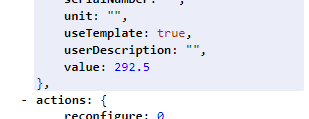# Transform raw value

hi Bill
is any way to transform raw value from the device?
example.
Fuckibaro HC3 pass WinDirection as simple com.fibaro.multilevelSensor without any measures
it’s look likeso my question is - is any way how I can re-calculate this value (“N”, “NNE”, “NE”, “ENE”, “E”, “ESE”, “SE”, “SSE”, “S”, “SSW”, “SW”, “WSW”, “W”, “WNW”, “NW”, “NNW”) and bind thise value instead of raw value.

plugin is ok, but have no use for =)

You could use DataTriggers along with RegEx to display custom values like N, NE, etc in a Label.

regex - no chance =)
it math operation, i thinking you know why =)

Not sure, understand the question right,… but for converting degree I use this:

``````function convertAngleToDirection(wind_angle) {
var windDir = ["N", "NO", "O", "SO", "S", "SW", "W", "NW", "N"];
var windx = parseInt((wind_angle + 23) / 45);
if (windx < windDir.length) {
return windDir[windx];
}
return "wind_angle";
}
``````

This I have integrated into code to display the direction.

@michapr thnx.
i know how calc =)
the main question how to call this code.
but i have a answer - no how +)